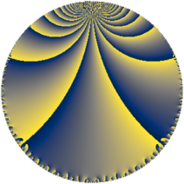# Properties

 Label 76.5.jLevel $76$ Weight $5$ Character orbit 76.j Rep. character $\chi_{76}(13,\cdot)$ Character field $\Q(\zeta_{18})$ Dimension $42$ Newform subspaces $1$ Sturm bound $50$ Trace bound $0$

# Learn more

## Defining parameters

 Level: $$N$$ $$=$$ $$76 = 2^{2} \cdot 19$$ Weight: $$k$$ $$=$$ $$5$$ Character orbit: $$[\chi]$$ $$=$$ 76.j (of order $$18$$ and degree $$6$$) Character conductor: $$\operatorname{cond}(\chi)$$ $$=$$ $$19$$ Character field: $$\Q(\zeta_{18})$$ Newform subspaces: $$1$$ Sturm bound: $$50$$ Trace bound: $$0$$

## Dimensions

The following table gives the dimensions of various subspaces of $$M_{5}(76, [\chi])$$.

Total New Old
Modular forms 258 42 216
Cusp forms 222 42 180
Eisenstein series 36 0 36

## Trace form

 $$42q + 12q^{3} - 45q^{7} - 84q^{9} + O(q^{10})$$ $$42q + 12q^{3} - 45q^{7} - 84q^{9} - 45q^{11} + 33q^{13} - 393q^{15} + 909q^{17} + 1242q^{19} + 1107q^{21} - 360q^{23} - 810q^{25} - 7056q^{27} - 2889q^{29} + 2808q^{31} + 10875q^{33} + 6741q^{35} - 3480q^{39} - 3060q^{41} - 8079q^{43} - 4320q^{45} - 2655q^{47} - 474q^{49} - 12222q^{51} - 6705q^{53} + 4623q^{55} - 8022q^{57} + 24309q^{59} + 7104q^{61} + 12063q^{63} + 25245q^{65} + 15573q^{67} - 10881q^{69} - 25506q^{71} + 3036q^{73} + 12924q^{77} - 16839q^{79} - 2208q^{81} - 6363q^{83} - 37890q^{85} - 21924q^{87} - 22644q^{89} + 17418q^{91} + 8184q^{93} - 82413q^{95} + 13383q^{97} + 23565q^{99} + O(q^{100})$$

## Decomposition of $$S_{5}^{\mathrm{new}}(76, [\chi])$$ into newform subspaces

Label Dim. $$A$$ Field CM Traces $q$-expansion
$$a_2$$ $$a_3$$ $$a_5$$ $$a_7$$
76.5.j.a $$42$$ $$7.856$$ None $$0$$ $$12$$ $$0$$ $$-45$$

## Decomposition of $$S_{5}^{\mathrm{old}}(76, [\chi])$$ into lower level spaces

$$S_{5}^{\mathrm{old}}(76, [\chi]) \cong$$ $$S_{5}^{\mathrm{new}}(19, [\chi])$$$$^{\oplus 3}$$$$\oplus$$$$S_{5}^{\mathrm{new}}(38, [\chi])$$$$^{\oplus 2}$$